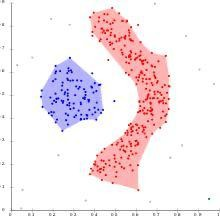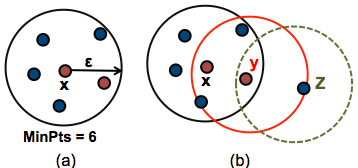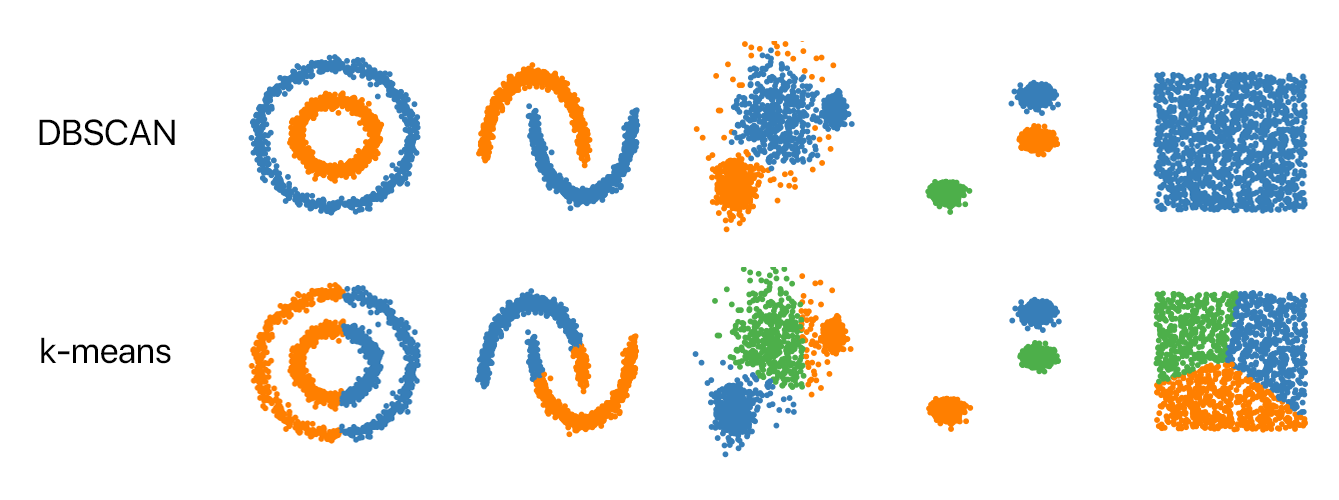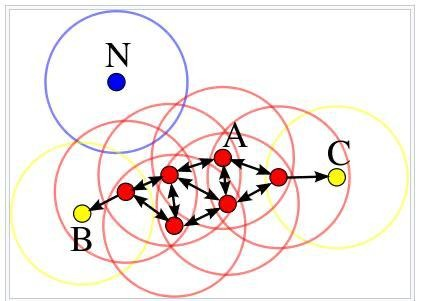CHIRAG GOYAL — Published On May 24, 2021 and Last Modified On June 24th, 2022

This article was published as a part of the Data Science Blogathon

## Introduction

DBSCAN(Density-Based Spatial Clustering Application with Noise), an unsupervised machine learning technique is one of the density-based clustering algorithms which is heavily used when we want to deal with the outliers and want the clusters of any random(arbitrary) shapes and sizes.

Therefore it becomes necessary for every aspiring Data Scientist and Machine Learning Engineer to have a good knowledge of this algorithm.

In this article, we will discuss the most important questions on the DBSCAN Clustering Algorithm which is helpful to get you a clear understanding of the algorithm, and also for Data Science Interviews, which covers its very fundamental level to complex concepts.

## 1. What is the DBSCAN Algorithm?

DBSCAN also known as Density-Based Spatial Clustering Application with Noise is an unsupervised machine learning algorithm that forms the clusters based upon the density of the data points or how close the data is.

As a result, the points which are outside the dense regions are excluded and considered as the noisy points or outliers. This characteristic of the DBSCAN algorithm makes it a perfect fit for outlier detection and making clusters of any random shapes and sizes.

This algorithm works on a parametric approach that uses two parameters i.e., eps(epsilon) and min_pts.

• eps: It represents the radius of the neighbourhoods around a data point x.
• min_pts: It is the minimum number of data points that we want in the neighbourhood of a particular point to define a cluster.## 2. Explain the DBSCAN Algorithm step by step.

The major steps followed during the DBSCAN algorithm are as follows:

Step-1: Decide the value of the parameters eps and min_pts.

Step-2: For each data point(x) present in the dataset:

• Compute its distance from all the other data points. If the distance is less than or equal to the value of epsilon(eps), then consider that point as a neighbour of x.
• If that data point(x) gets the count of its neighbour greater than or equal to min_pts, then mark it as a core point or as visited.

Step-3: For each core point, if it is not already assigned to a cluster then create a new cluster. Further, all the neighbouring points are recursively determined and are assigned the same cluster as that of the core point

Step-4: Repeat the above steps until all the points are visited.

## 3. What are Density-Based models?

Density-based models are the types of models that try to search the data space for the areas of the varied density of data points. It separates different density regions by assigning the data points within these regions in the same cluster.

For Example,

Density-based clustering: Creates clusters according to the density measurement since clusters have a higher density than the rest of the dataset which is observed by calculating the density in the data space.

## 4. Which is the most widely used Density-based Clustering Algorithm?

The most widely used density-based algorithm is Density-Based Spatial Clustering of Applications with Noise (DBSCAN), which uses the idea of density reachability and density connectivity.

## 5. What is Density-based Clustering?

Density-Based Clustering is an unsupervised machine learning method that identifies different groups or clusters in the data space. These clustering techniques are based on the concept that a cluster in the data space is a contiguous region of high point density, separated from other such clusters by contiguous regions of low point density.

Partition-based(K-means) and Hierarchical clustering techniques are highly efficient with normal-shaped clusters while density-based techniques are efficient in arbitrary-shaped clusters or detecting outliers.

## 6. List out the Input parameters given to the DBSCAN Algorithm.

The DBSCAN algorithm uses the following two user-defined as the input parameters for clustering:

• Epsilon (eps): It is defined as the maximum distance between two points which are considered as neighbouring points as well as can be viewed as the radius around each point.
• Minimum Points (min_samples or min_pts): This defines the minimum number of neighbouring points that a given point needs to be considered a core data point including the point itself. In general terms, it can be considered as the minimum number of observations that should be around that point within that radius.

For example, if we set the parameter min_pts to 4, then a given point needs to have 3 or more neighbouring data points to become a core data point.

Moreover, if the minimum number of points meet the epsilon distance requirement then they are considered as a cluster.

## 7. How can we interpret the parameters “eps” and “min_pts” in high dimensions for the DBSCAN Algorithm?

In the case of higher dimensions, the parameter epsilon can be viewed as the radius of that hypersphere and min_pts as the minimum number of data points required inside that hypersphere.

## 8. What are density reachability and density connectivity?

Reachability in terms of density sets up a point to be reachable from another if it lies within a distance of epsilon i.e, the radius of the circle from it.

On the contrary, Connectivity involves a transitivity based chaining-approach to determine whether points are located in a particular cluster.

For example, Two points x and y points could be connected if x->r->s->t->y where a->b implies that b is in the neighbourhood of a.

## 9. How does the epsilon value affect the DBSCAN Clustering Algorithm?

The DBSCAN Algorithm is sensitive to the choice of epsilon. When we have clusters with varying densities, then two cases arise i.e.,

If epsilon is too small: In such cases, we define the sparser clusters as noise i.e, result in the elimination of sparse clusters as outliers.

If epsilon is too large: In such cases, the denser clusters may be merged together, which gives the incorrect clusters.

## 10. Is the DBSCAN Algorithm sensitive to the values of the parameters?

Yes, the DBSCAN algorithm is very sensitive to the values of epsilon and min_pts. Therefore, it is crucial to understand how to choose the values of epsilon and min_pts. A minor variation in these values can change the results significantly produced by the DBSCAN algorithm.

## 11. How is the parameter “Distance-function” estimated in the DBSCAN Algorithm?

The choice of distance function is tightly linked to the choice of epsilon and has a major impact on the outcomes. In general, it will be necessary to first identify a reasonable measure of similarity for the data set, before the parameter epsilon can be chosen.

There is no estimation for this parameter, but the distance functions need to be chosen appropriately based on the nature of the data set.

DBSCAN uses Euclidean distance by default, although other methods can also be used.

For Example, For geographical data, we use the great circle distance.

## 12. Explain the following terms related to DBSCAN Algorithm:

– Direct Density Reachable

– Density Reachable

– Density Connected

Direct density reachable: A point is called direct density reachable if it has a core point in its neighbourhood.

Density Reachable: A point is known as density reachable from another point if they are connected through a series of core points.

Density Connected: Two points are called density connected if there is a core point that is density reachable from both points.

## 13. How many types of points do we get after applying a DBSCAN Algorithm to a particular dataset?

We get three types of points upon applying a DBSCAN algorithm to a particular dataset – Core point, Border point, and noise point.

• Core Point: A data point is considered to be a core point if it has a minimum number of neighbouring data points (min_pts) at an epsilon distance from it. These min_pts include the original data points also.
• Border Point: A data point that has less than the minimum number of data points needed but has at least one core point in the neighbourhood.
• Noise Point: A data point that is not a core point or a border point is considered noise or an outlier.

In other words, we can say that if the number of neighbourhood points around x is greater or equal to MinPts then x is treated as a core point, if the neighbourhood points around x are less than MinPts but are close to a core point then x is treated as a border point. If x is neither a core point nor a border point then x is considered as a noisy point or an outlier.

## 14. How is the parameter “eps” estimated in the DBSCAN Algorithm?

The value for epsilon can then be chosen by using a K-distance Graph, which is a plot of the distance to the k = min_pts – 1 nearest neighbour ordered from the maximum to the minimum value. Then, choose the values of epsilon where this plot shows an “elbow”.

• Too small epsilon: If epsilon is chosen much too small, then a large part of the data will not be clustered.
• Too large epsilon: If epsilon is chosen much too large, then clusters will merge and the majority of objects will be in the same cluster.

Therefore in general smaller values of epsilon are preferable and usually, only a small fraction of points remain within this distance of each other.

## 15. After applying the DBSCAN Algorithm on a dataset, we get the following clusters(as shown below in the figure). Identify the core point, border point, and noise point.Core Point: The data point x is the core point since it has at least min_pts (n) within epsilon (eps) distance.

Border Point: The data point y is the border point since it has at least one core point within epsilon (eps) distance and lower than min_pts (n) within epsilon (eps) distance from it.

Noise Point: The data point z is the noise point since it has no core points within epsilon (eps) distance.

## 16. Why does there arise a need for DBSCAN when we already have other clustering Algorithms?

Partitioning methods like K-means, PAM clustering, etc, and hierarchical clustering work for finding spherical-shaped clusters or convex clusters i.e, they are suitable only for compact and well-separated clusters and are also critically affected by the presence of noise and outliers in the data. Since real-life data often contain various irregularities such as:

• Clusters can be of arbitrary shape.
• Data may contain noisy points.

To overcome such problems DBSCAN is used as it produces more reasonable results than k-means across a variety of different distributions. We can elucidate this through the following fact:## 17. What is the time complexity of the DBSCAN Clustering Algorithm?

The different complexities of the algorithm are(N= no of data points) as follows:

Best Case: If we use a spatial indexing system to store the dataset like kd-tree or r-tree such that neighbourhood queries are executed in logarithmic time, then we get O(N log N) runtime complexity.

Worst Case: Without the use of index structure or on degenerated data the worst-case run time complexity remains O(N2).

Average Case: It is the same as the best/worst case depending on data and implementation of the algorithm.

## 18. How is the parameter “min_pts” estimated in the DBSCAN Algorithm?

As a rule of thumb, the minimum bound of the parameter “min_pts” can be computed from the number of dimensions D in the data set, as min_pts ≥ D + 1.

Case-1: The low value of min-Pts i.e, min_pts=1 does not make sense, as then every point on its own will already be a cluster.

Case-2: When min_pts ≤ 2, the result will be the same as that of the hierarchical clustering with the metric as a single link, having dendrogram cut at a height of epsilon.

Therefore, min_pts must be chosen at least 3.

However, larger values of min_pts are usually better when the data sets are having noise and as a result, it will yield more significant clusters.

Generally, as a rule of thumb min_pts = 2*D can be used.

To choose larger values, it may be necessary that the:

• Data is very large
• The Data is noisy i.e, contains outliers
• Data contains many duplicates

## 19. What are the advantages of the DBSCAN density-based Clustering Algorithm?

Some of the advantages of the DBSCAN algorithm are as follows:

1. It does not need a predefined number of clusters i.e, not require an initial specification of the number of clusters.

2. Basically, clusters can be of any random shape and size, including non-spherical ones.

3. It is able to identify noise data, popularly known as outliers.

4. Unlike K means, In DBSCAN the user does not give the number of clusters to be generated as input to the algorithm.

5. DBSCAN can find any shape of clusters.

6. Also, the cluster doesn’t have to be circular.

7. Density-based clustering algorithms don’t include the outliers in any of the clusters. Outliers were considered as noise while clustering and hence they will be eliminated from the cluster after the algorithm completion.

## 20. What are the disadvantages of the DBSCAN density-based Clustering Algorithm?

Some of the disadvantages of the DBSCAN algorithm are as follows:

1. DBSCAN clustering will fail when there are no density drops between clusters.

2. It seems to be difficult to detect outlier or noisy points if there is a variation in the density of the clusters.

3. It is sensitive to parameters i.e. it’s hard to determine the correct set of parameters.

4. Distance metric also plays a vital role in the quality of the DBSCAN algorithm

5. With high dimensional data, it does not give effective clusters

6. Not partitionable for multiprocessor systems.

## Discussion Problem

Below given is an image in which the value of min_pts is 4. Accordingly, find the value of (x+y+z+t).where,

x = Sum of the alphabet positions according to the English dictionary of the core points

y = Sum of the alphabet positions according to the English dictionary of the noisy points

z = Max(alphabet position according to the English dictionary of the border points)

t = Number of border points

For Example, In the series of the alphabet, D’s position is 4, T’s position is 20, etc.

Try to solve the Practice Question and answer it in the comment section below.

For any further queries feel free to contact me.

## End Notes

I hope you enjoyed the questions and were able to test your knowledge about DBSCAN Clustering Algorithm.

If you liked this and want to know more, go visit my other articles on Data Science and Machine Learning by clicking on the Link

Something not mentioned or want to share your thoughts? Feel free to comment below And I’ll get back to you.

## Chirag Goyal

Currently, I pursuing my Bachelor of Technology (B.Tech) in Computer Science and Engineering from the Indian Institute of Technology Jodhpur(IITJ). I am very enthusiastic about Machine learning, Deep Learning, and Artificial Intelligence.

The media shown in this article are not owned by Analytics Vidhya and is used at the Author’s discretion.